Next: Ginzburg-Landau Theory Up: Magnetic Properties of Conventional Superconductors Previous: The London Penetration Depth

## The Coherence Length (Pippard's Equation)

The London equations whose derivation appears elsewhere (see  for instance), are local equations (i.e. they relate the current density at a point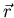to the vector potential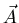at the same point), and hence define the superconducting properties as such. However, early discrepancies between experimental estimations of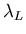(0) (the penetration depth at zero temperature) for certain conventional superconductors, and those predicted by Eq. (2.1) led Pippard [13,15] in 1950 to introduce non-local effects into the London equations. Spatial changes of quantities such as ns in a superconductor may only occur on a finite length scale, the coherence length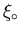, and not over arbitrarily small distances. That is to say, whenever ns is varying in space its value may change significantly over distances of order. Thus the coherence length defines the intrinsic nonlocality of the superconducting state. Using the uncertainty principle, Pippard estimated the coherence length for a pure metal to be [17,18]: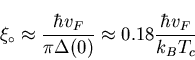(4)

where kB is Boltzmann's constant, and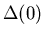is the energy gap that forms at the Fermi surface in the superconducting state at absolute zero. This will be discussed in more detail later.

In the London model, the local value of the magnetic flux density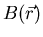and the supercurrent density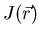are assumed to vary slowly in space on a length scale, and have negligible variation over distances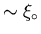. In Fig. 2.2, the superconducting carrier density nsjumps discontinuously from zero to a maximum value at the sample surface. As already mentioned, ns is expected to make a significant change like this only over a distance on the order of. Thus in Fig. 2.2,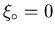, so that one would anticipate an exponential screening of the magnetic field in a real superconductor only when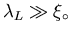. Indeed, the London model is valid only for.

In a type-I superconductor, the magnetic penetration depthis much less than the coherence length, so that ns does not reach its maximum value near the surface. That is to say, not all of the electrons within a thicknessfrom the surface of the superconductor contribute to the screening currents. Consequently, the penetration of the magnetic field does not follow the exponential form of Eq. (2.2) until one ventures an appreciable distance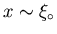into the superconductor. Thus the usual London model is inadequate in describing type-I superconductors where,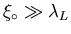. A modification of the London equations which gives the magnetic flux and supercurrent densities a more rapid variation in space, predicts the actual penetration depth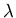in a type-I superconductor to be :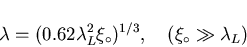(5)

whereis larger than, but much smaller than.Next: Ginzburg-Landau Theory Up: Magnetic Properties of Conventional Superconductors Previous: The London Penetration Depth
Jess H. Brewer
2001-09-28